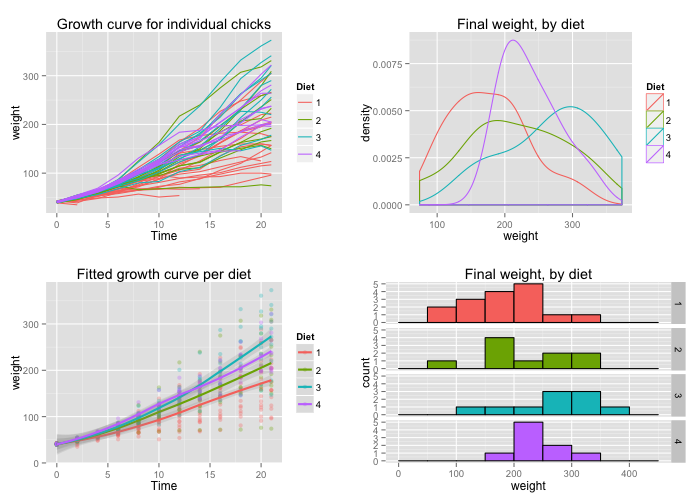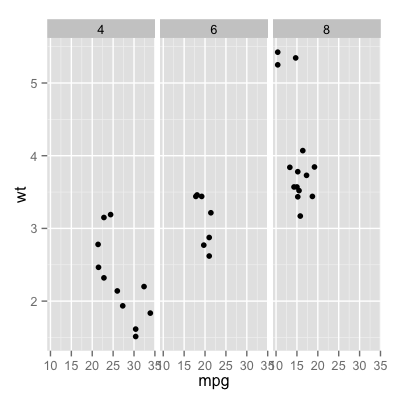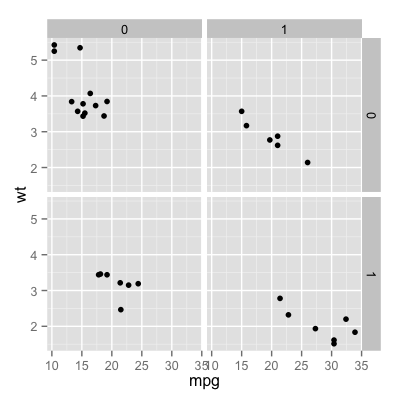The easy way is to use the multiplot function to put multiple graphs on one page, defined at the bottom of this page. If it isn’t suitable for your needs, you can copy and modify it.

## Problem

You want to put multiple graphs on one page.

## Solution-1

use the multiplot function

### plots and store

First, set up the plots and store them, but don’t render them yet. The details of these plots aren’t important; all you need to do is store the plot objects in variables.

### multiplot function

This is the definition of multiplot. It can take any number of plot objects as arguments, or if it can take a list of plot objects passed to plotlist.

### multiplot

Once the plot objects are set up, we can render them with multiplot. This will make two columns of graphs:## Solution-2

facet_grid## Solution-3

grid.arrange0%